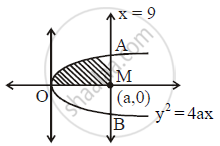Share

# Find the area bounded by the curve y^2 = 4ax, x-axis and the lines x = 0 and x = a. - Mathematics and Statistics

#### Question

Find the area bounded by the curve y2 = 4axx-axis and the lines x = 0 and x = a.

#### SolutionArea bounded = int_0^aydx

=int_0^asqrt(4ax)dx

=2sqrtaint_0^ax^(1/2)dx

=2sqrta[(x^(1/2+1))/(1/2+1)]_0^a

=2sqrtaxx2/3xxa^(3/2)

=4/3a^(1/2+3/2)

=4/3a^2

Is there an error in this question or solution?

#### APPEARS IN

2015-2016 (March) (with solutions)
Question 4.2.3 | 2.00 marks
2014-2015 (October) (with solutions)
Question 4.2.2 | 2.00 marks

#### Video TutorialsVIEW ALL 

Solution Find the area bounded by the curve y^2 = 4ax, x-axis and the lines x = 0 and x = a. Concept: Area of the Region Bounded by a Curve and a Line.
S